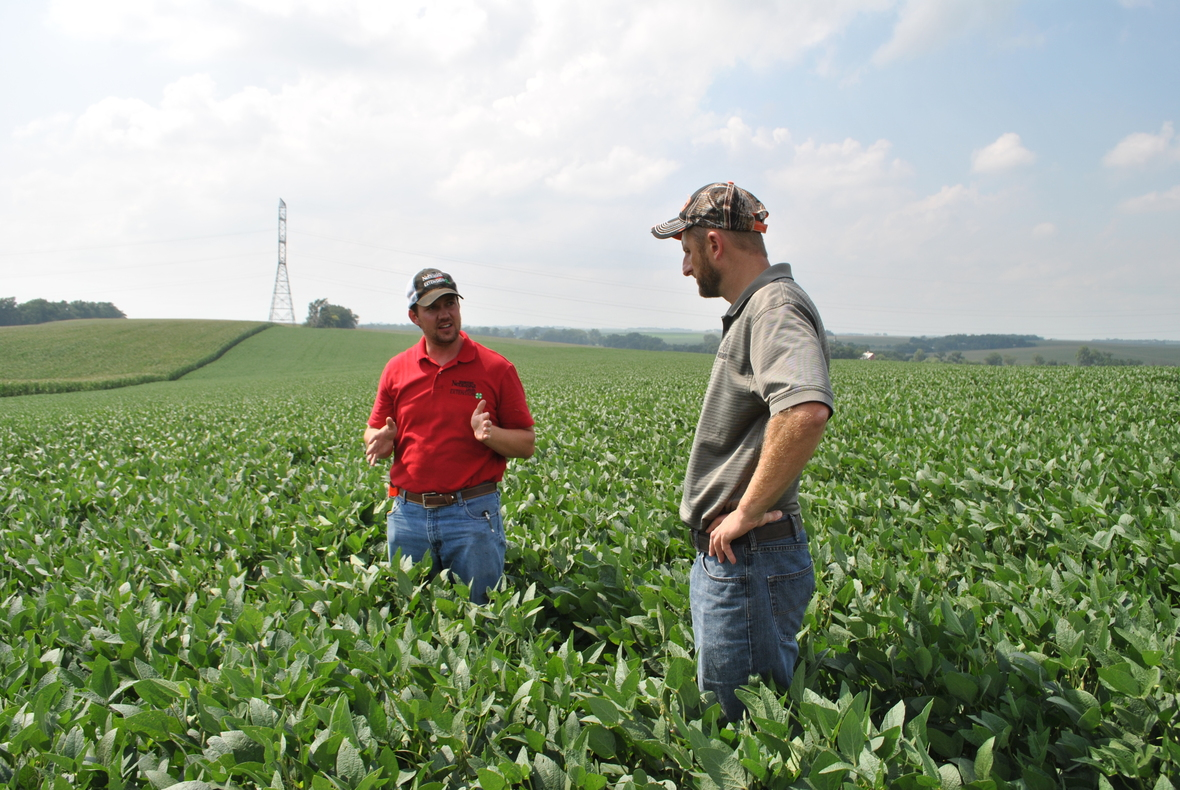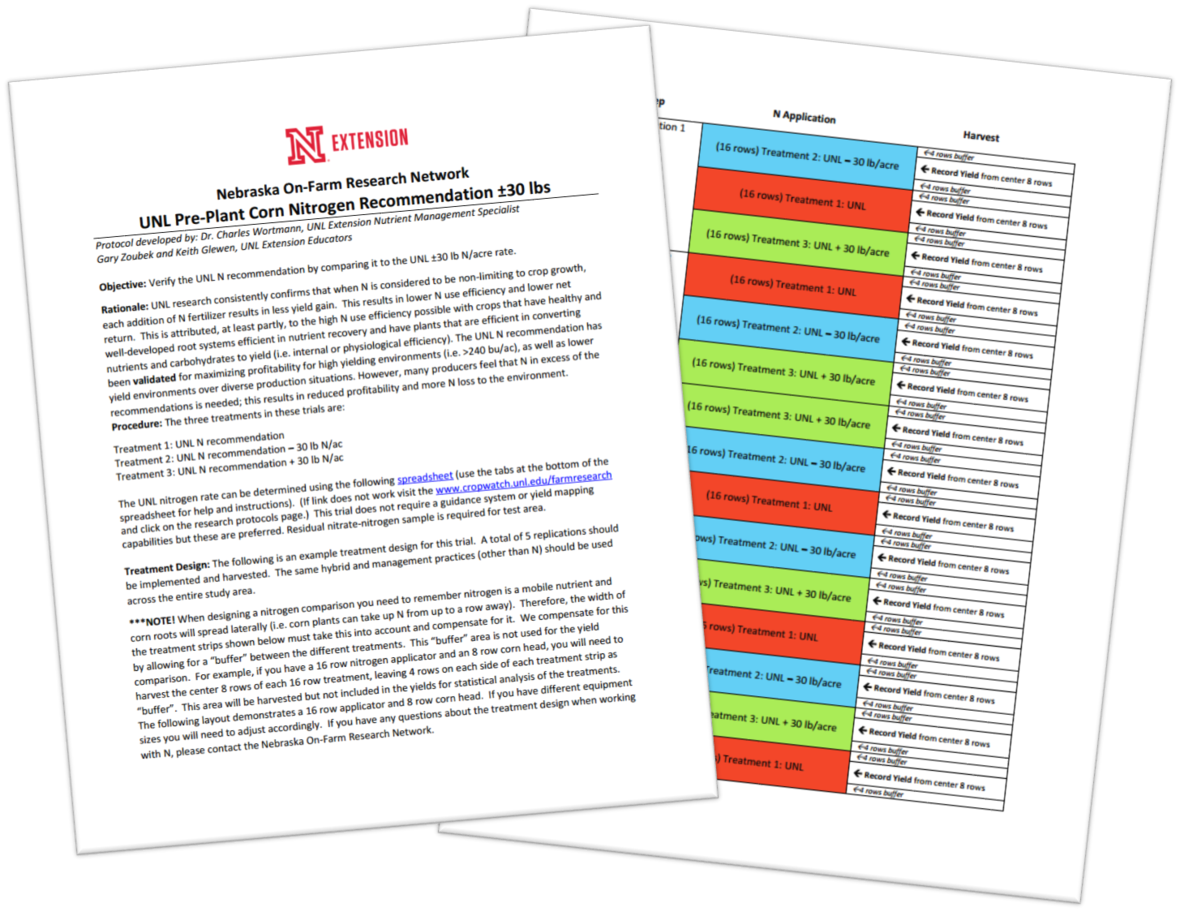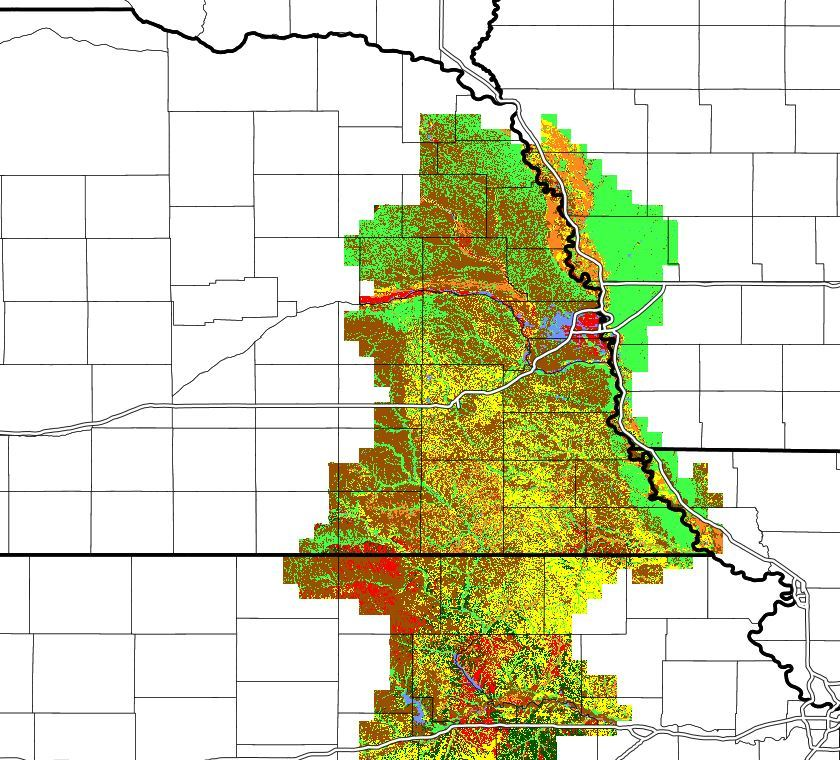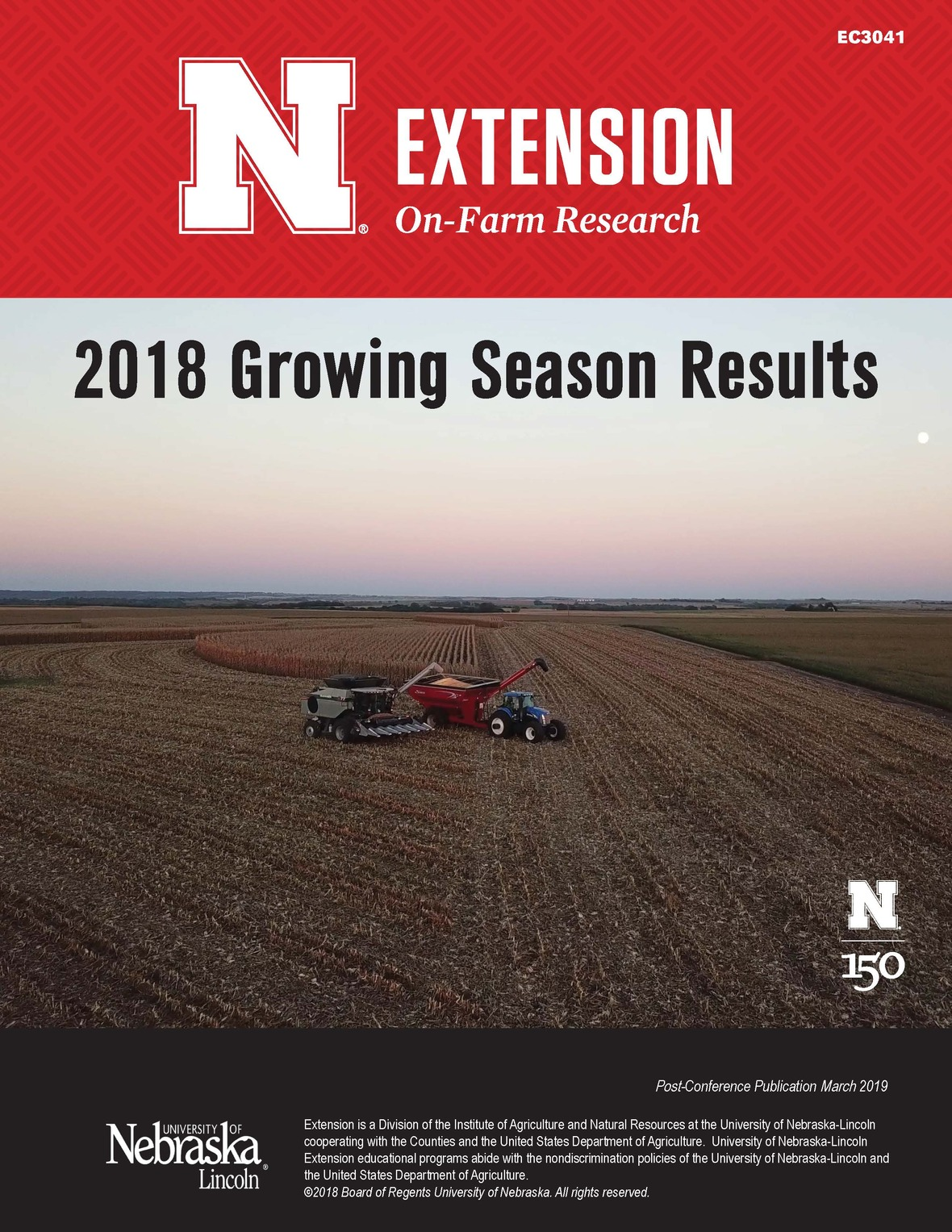/* styles */ April 2019

## Have a research idea? We're here to help!/* styles */ Do you have an idea about how to improve your farming operation? On-farm research is a great way to test that idea on a smaller scale before implementing the change on your whole operation. There is still time to plan your 2019 on-farm research projects. Nebraska Extension Educators can help you: 1. Set up your experiment to get reliable results 2. Assist with in-season data collection 3. Analyze yield data 4. Provide you with a summary report of your study
 table div table+table+table+table+table+table+table div table{width:100%;padding:0}table div table+table+table+table+table+table+table div table img{width:96.23%;padding:0;float:none}table div table+table+table+table+table+table+table div table td{width:100%;padding:0 1.88% 18px}/* styles */## Need an idea for research? Browse ready-to-use protocols online./* styles */ We have lots of ready-to-use research protocols on our website, and we will be adding more in the upcoming weeks. Protocols are already developed for many topics including cover crop interseeding, fungicide application on corn, soybean planting rates, corn planting rates, soybean planting date, soybean maturity group comparison, various nitrogen management techniques, and more. All protocols have a sample study map, can be downloaded, and are printer friendly.
 table div table+table+table+table+table+table+table+table+table+table+table+table div table{width:100%;padding:0}table div table+table+table+table+table+table+table+table+table+table+table+table div table img{width:96.23%;padding:0;float:none}table div table+table+table+table+table+table+table+table+table+table+table+table div table td{width:100%;padding:0 1.88% 18px}/* styles */## Featured Study Opportunity: Soybean Yield Gap ResearchThe shaded areas (red, brown, orange, yellow, green, blue) indicate the target areas where this study can be conducted.
 /* styles */ The Nebraska On-Farm Research Network (NOFRN) is seeking 20 farmers to participate in a study of practices affecting the soybean yield gap. That's the difference between current farm yield and potential yield as determined by climate, soil, and genetics. An analysis of survey responses from over 2000 soybean producers indicated a 20%-30% yield gap for soybean. The analysis also revealed a number of agronomic practices that, for a given soil-climate context, could be fine-tuned to close the gap and improve profit from soybean. In Nebraska, three practices have been identified as being important for improving yield and producer profit. These practices relate to planting date, seeding rate, and the use of foliar fungicides and insecticides. The study is seeking 20 growers in eastern Nebraska to test "improved" practices versus "baseline" practices in 2019 and 20 again for 2020. Participating growers will be provided some monetary compensation. To participate, contact Nebraska Educators and NOFRN Co-coordinators Keith Glewen (402-624-8030, kglewen1@unl.edu) or Laura Thompson (402-245-2224, laura.thompson@unl.edu), or your local extension educator.
 table div table+table+table+table+table+table+table+table+table+table+table+table+table+table+table+table+table div table{width:100%;padding:0}table div table+table+table+table+table+table+table+table+table+table+table+table+table+table+table+table+table div table img{width:96.23%;padding:0;float:none}table div table+table+table+table+table+table+table+table+table+table+table+table+table+table+table+table+table div table td{width:100%;padding:0 1.88% 18px}/* styles */## 2018 On-farm research results available onlineThere are two ways to access the 2018 growing season research results.

1. Download a copy of the 2018 Growing Season Results book as a PDF.
 1 Download a copy of the 2018 Growing Season Results book as a PDF.
 /* styles */ 2.Search the Results Finder database. This database contains over 800 on-farm research studies, including those conducted in 2018.
 table div table+table+table+table+table+table+table+table+table+table+table+table+table+table+table+table+table+table+table+table+table+table+table+table div table{width:100%;padding:0}table div table+table+table+table+table+table+table+table+table+table+table+table+table+table+table+table+table+table+table+table+table+table+table+table div table img{width:96.23%;padding:0;float:none}table div table+table+table+table+table+table+table+table+table+table+table+table+table+table+table+table+table+table+table+table+table+table+table+table div table td{width:100%;padding:0 1.88% 18px}/* styles */table.module-24{width:0%;padding:0}table div table+table+table+table+table+table+table+table+table+table+table+table+table+table+table+table+table+table+table+table+table+table+table+table+table div table{width:0%;float:none;margin-left:auto;margin-right:auto;padding:0}table div table+table+table+table+table+table+table+table+table+table+table+table+table+table+table+table+table+table+table+table+table+table+table+table+table div table a{border:0 none;text-decoration:none}table div table+table+table+table+table+table+table+table+table+table+table+table+table+table+table+table+table+table+table+table+table+table+table+table+table div table img{width:100%!important;border:0 none;text-decoration:none}table div table+table+table+table+table+table+table+table+table+table+table+table+table+table+table+table+table+table+table+table+table+table+table+table+table div table td{width:100%;padding:0}/* styles */
 /* styles */
 Like   Tweet   Pin   +1   in